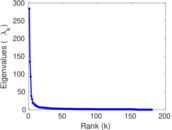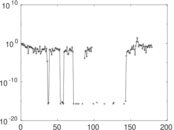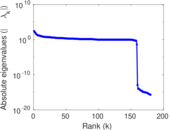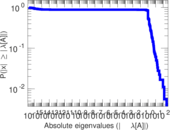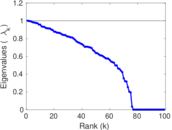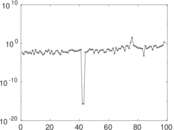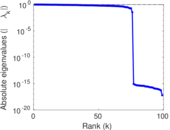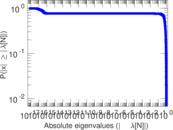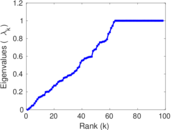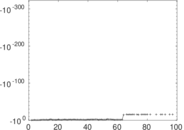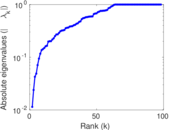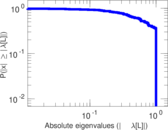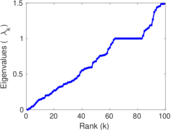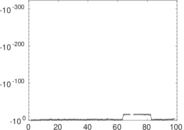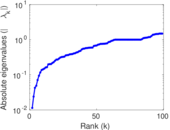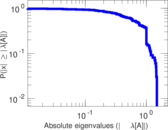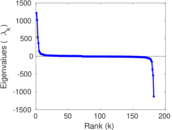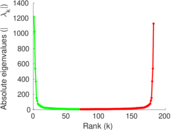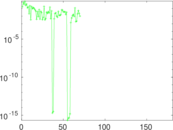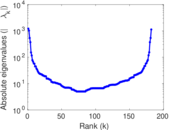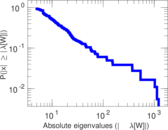# Wikiquote edits (kn)

This is the bipartite edit network of the Kannada Wikiquote. It contains users and pages from the Kannada Wikiquote, connected by edit events. Each edge represents an edit. The dataset includes the timestamp of each edit.

 Code `qkn` Internal name `edit-knwikiquote` Name Wikiquote edits (kn) Data source http://dumps.wikimedia.org/ AvailabilityDataset is available for download Consistency checkDataset passed all tests Category Authorship network Dataset timestamp 2017-10-20 Node meaning User, article Edge meaning Edit Network formatBipartite, undirected Edge typeUnweighted, multiple edges Temporal dataEdges are annotated with timestamps

## Statistics

 Size n = 1,126 Left size n1 = 182 Right size n2 = 944 Volume m = 3,075 Unique edge count m̿ = 1,201 Wedge count s = 77,245 Claw count z = 6,499,694 Cross count x = 459,274,619 Square count q = 1,852 4-Tour count T4 = 326,386 Maximum degree dmax = 1,425 Maximum left degree d1max = 1,425 Maximum right degree d2max = 205 Average degree d = 5.461 81 Average left degree d1 = 16.895 6 Average right degree d2 = 3.257 42 Fill p = 0.006 990 36 Average edge multiplicity m̃ = 2.560 37 Size of LCC N = 887 Diameter δ = 17 50-Percentile effective diameter δ0.5 = 3.814 39 90-Percentile effective diameter δ0.9 = 7.327 13 Median distance δM = 4 Mean distance δm = 4.693 74 Gini coefficient G = 0.760 575 Balanced inequality ratio P = 0.195 610 Left balanced inequality ratio P1 = 0.125 528 Right balanced inequality ratio P2 = 0.258 862 Relative edge distribution entropy Her = 0.817 518 Power law exponent γ = 5.066 20 Tail power law exponent γt = 2.631 00 Tail power law exponent with p γ3 = 2.631 00 p-value p = 0.000 00 Left tail power law exponent with p γ3,1 = 1.881 00 Left p-value p1 = 0.787 000 Right tail power law exponent with p γ3,2 = 3.281 00 Right p-value p2 = 0.720 000 Degree assortativity ρ = −0.238 690 Degree assortativity p-value pρ = 5.082 15 × 10−17 Spectral norm α = 284.235 Algebraic connectivity a = 0.011 403 4 Spectral separation |λ1[A] / λ2[A]| = 2.108 10 Controllability C = 793 Relative controllability Cr = 0.713 771

## Plots

### Fruchterman–Reingold graph drawing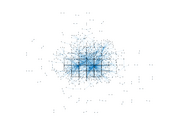### Degree distribution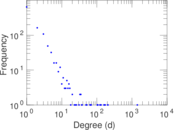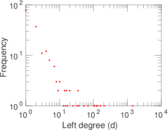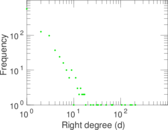### Cumulative degree distribution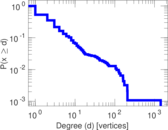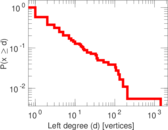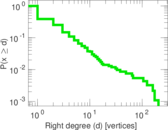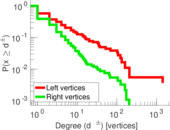### Lorenz curve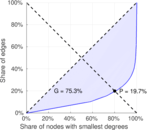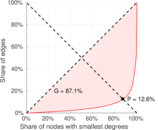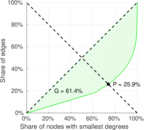### Spectral distribution of the adjacency matrix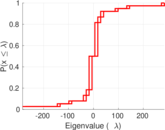### Spectral distribution of the normalized adjacency matrix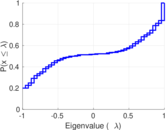### Spectral distribution of the Laplacian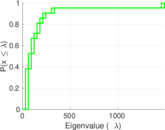### Spectral graph drawing based on the adjacency matrix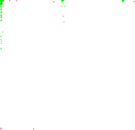### Spectral graph drawing based on the Laplacian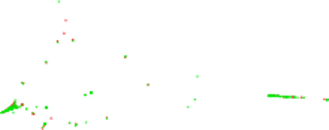### Spectral graph drawing based on the normalized adjacency matrix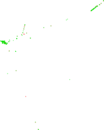### Degree assortativity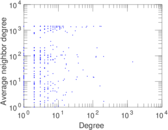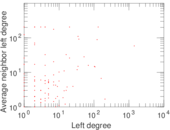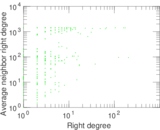### Zipf plot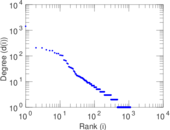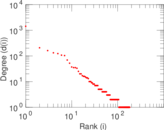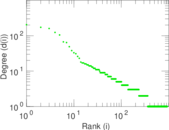### Hop distribution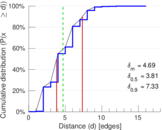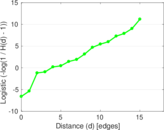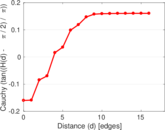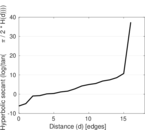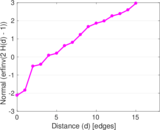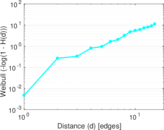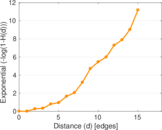### Double Laplacian graph drawing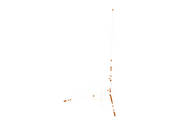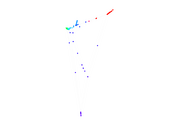### Delaunay graph drawing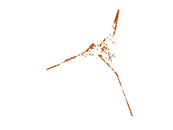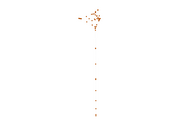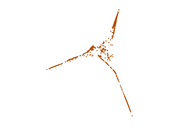### Edge weight/multiplicity distribution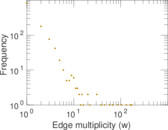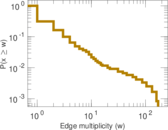### Temporal distribution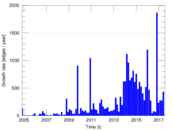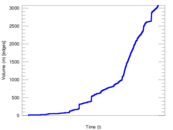### Temporal hop distribution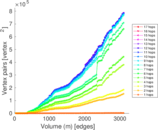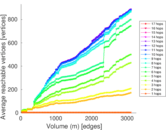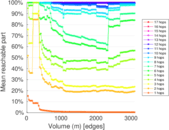### Diameter/density evolution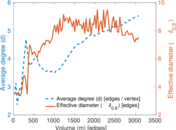### Matrix decompositions plots